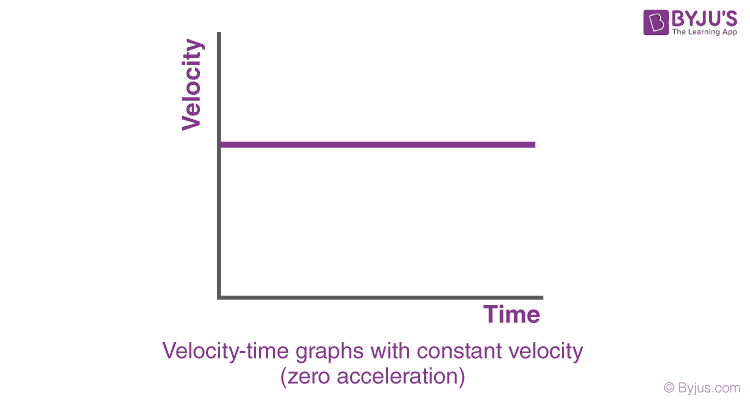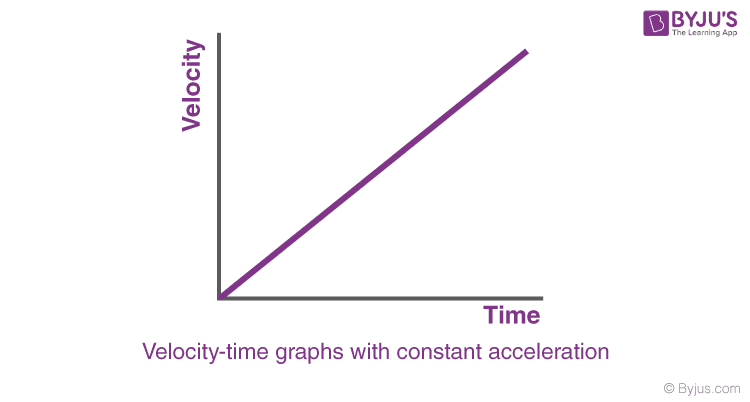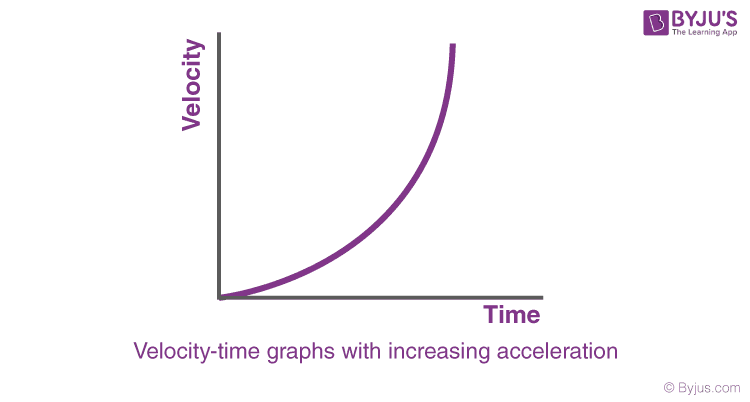# Velocity-Time Graphs

We have learnt what velocity is. Now, we will learn about the Velocity Time Graphs.

## Velocity-Time Graphs

To draw velocity time graphs, we will use the three equations of motion.

### Case 1: Velocity-time graphs with constant velocity (zero acceleration)

When the velocity is constant, the velocity time graph, with Y-axis denoting velocity and X-axis denoting time, will be like:As clear from the graph, the velocity is constant (c) throughout the time interval. No particles of matter how much the time changes, the velocity will be c at every instant. In this case, we have taken the initial velocity to be positive. The graph will be different if the initial velocity is negative.

Example: If the acceleration of a particle is zero (0), and velocity is constantly said 5 m/s at t =0, then it will remain constant throughout the time.

### Case 2: Velocity-time graphs with constant acceleration

When the acceleration is constant (positive), and the initial velocity of the particle is zero, the velocity of the particle will increase linearly as predicted by the equation:

v = u + at

Since u = 0

v = atAs shown in the figure, the velocity of the particle will increase linearly with respect to time. The slope of the graph will give the magnitude of acceleration.

Example: If the acceleration of a particle is constant (k) and is positive, the initial velocity is zero, and then the velocity increases linearly. The slope of the velocity-time graph will give the acceleration.

## Case 3: Velocity-time graphs with increasing acceleration

When the acceleration is increasing with time, the velocity-time graph will be a curve as predicted from the equation:
v = u + at
Since u = 0
v= at
Since acceleration is a function of time, the velocity-time graph will be a curve.
Note: Since the acceleration is continuously increasing with time, the magnitude of the slope will also continuously increase with time.Example: If the acceleration of a particle is a function of time and the initial velocity is zero, the velocity-time graph will be a curve. The slope of the velocity-time graph at any instant (at a certain time) will give the acceleration at that time.

Case 3 was just the case of increasing acceleration. There will be a different graph when the acceleration is decreasing with time. To learn more about velocity-time graphs, join BYJU’S.

Test Your Knowledge On Vt Graphs!